## brace matching in vim, regardless of how it is formatted?

August 31, 2016 C/C++ development and debugging. ,

DB2 functions were usually formatted with the brace on the leading line like so:

size_t table_count( T * table )
{
size_t count = 0 ;
....
}


For such code, typing [[ in vim anywhere from somewhere in the function text would take you to the beginning of the function. It has always annoyed me that this key sequence didn’t work for functions formatted without the leading { in the first column, such as

size_t table_count( T * table ) {
size_t count = 0 ;
....
}


Having my handy [[ command sequence take me to the first line of the file is pretty annoying, enough that I looked up the way to do what I want. A key sequence that does part of this job is:

[{

This takes you to the outermost ending position of the current scope, and you can use % to get to the beginning of that scope. You can repeat this as many times as necessary, until you get the outermost scope.

Is there a better way to go directly to the outermost scope directly, regardless of how the function happens to be formatted?

## New home office and network setup

August 30, 2016 Uncategorized

I’ve just bought a nice new desk for my home office:When I assembled the new desk, I took the opportunity to refine my home network setup. In this post I’ll walk through that setup.

I’ve got the router in the living room, along with the two NUC6i’s that I use for my lzlabs development work: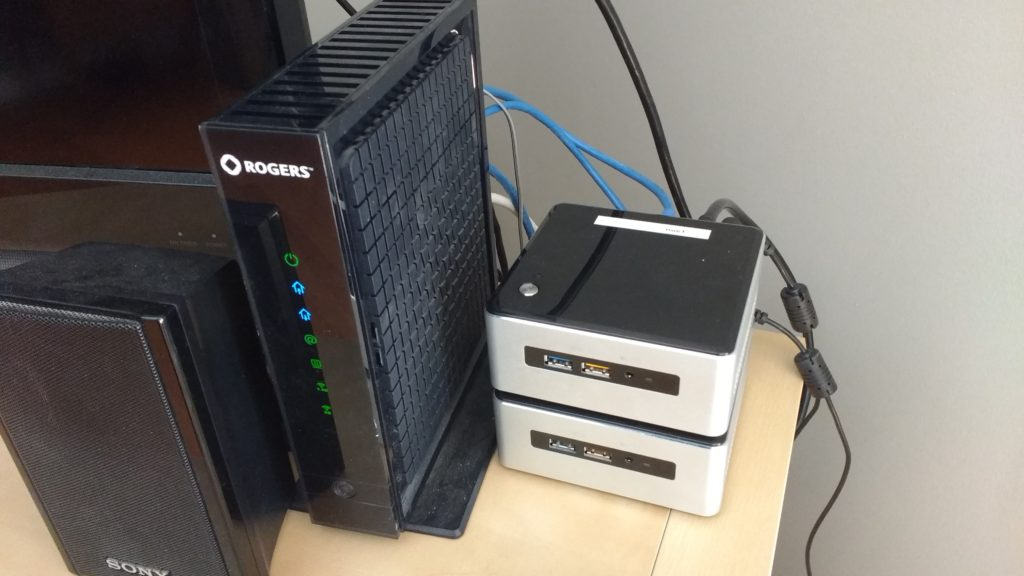I run the NUCs headless, using ssh and command line access for my work. For those times that I need to refresh the install image and need a display, I also have them HDMI connected to the living room TV, and direct connected with short ethernet cables to the router: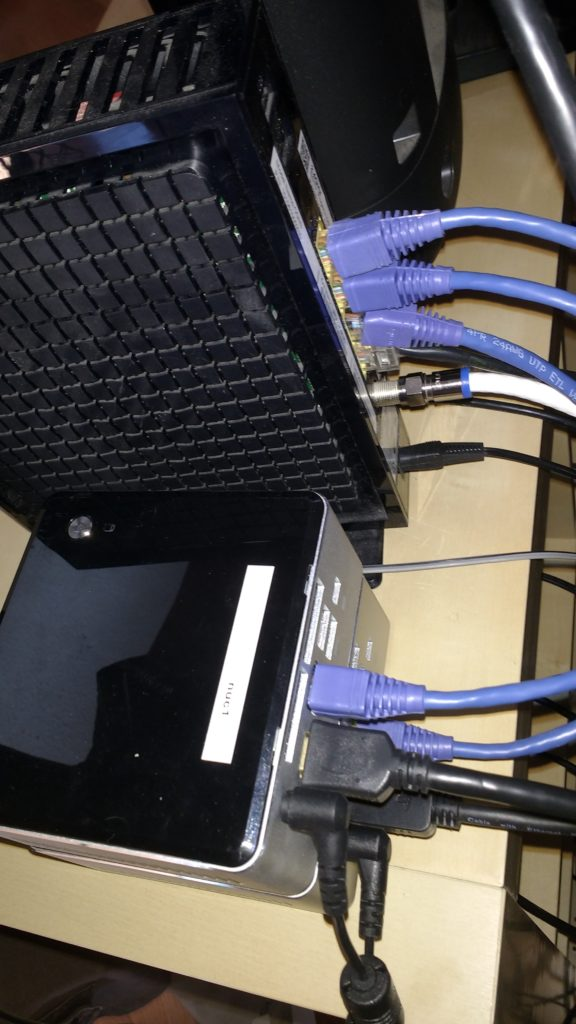The router is also directly connected to the PS3 (for netflix), and then runs through some ethernet lines that I fished into the basement, before some of the basement finishing work we did just after we moved in. All the lines I have going into the basement terminate in a patch panel: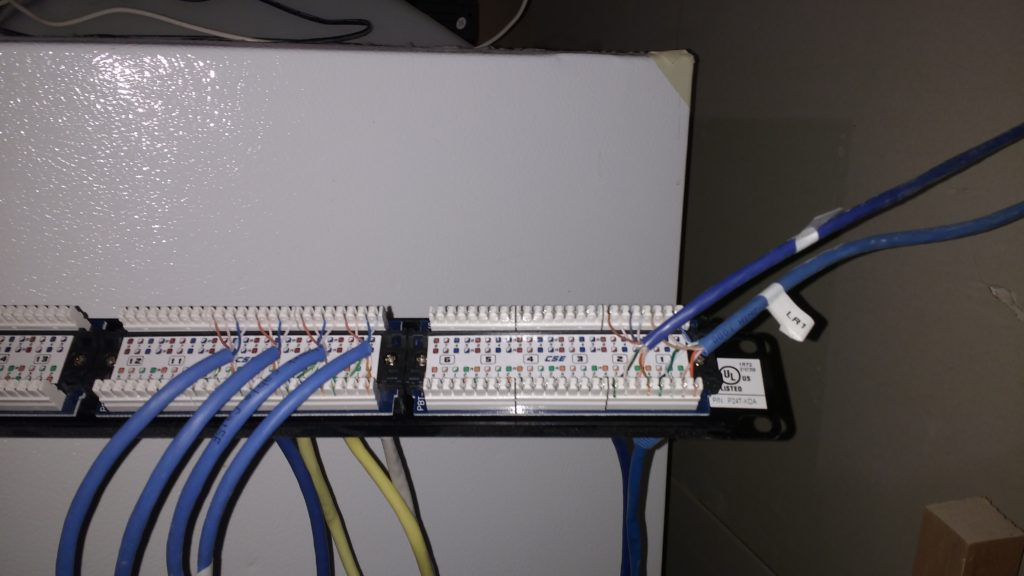Does a patch panel sound like overkill? Yes, it probably is. However, I’ve got a pile of roughed in ethernet lines to the kids rooms and the rec room that I’ll eventually fish into the electrical-panel/laundry/network room: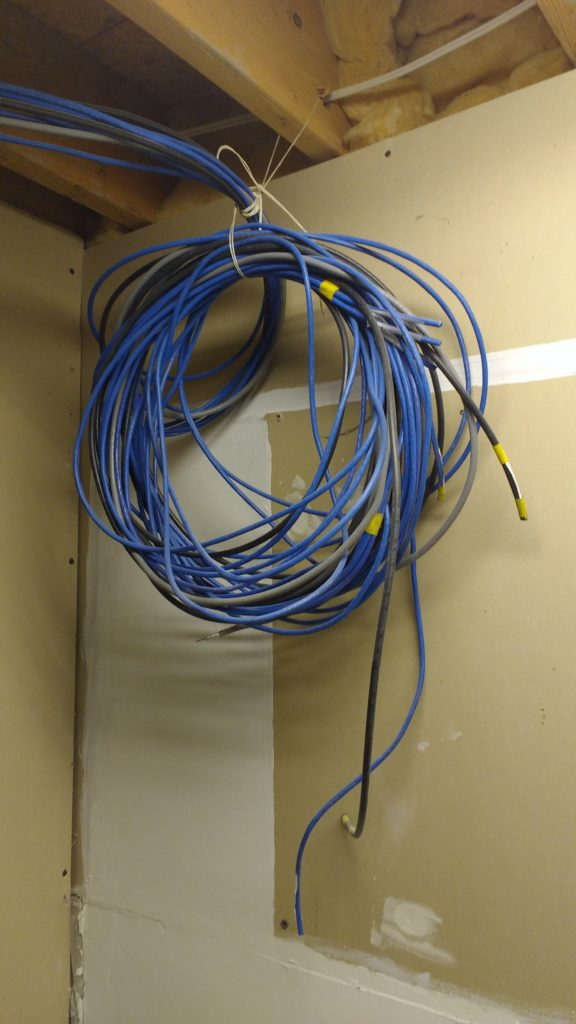I’d like to also eventually fish some ethernet lines into the upstairs space (i.e. for netflix run off a blue-ray player that sits mostly unused in the master bedroom), but that’s a harder fishing job, and I haven’t done it yet. From the front of the patch panel things go off to the router: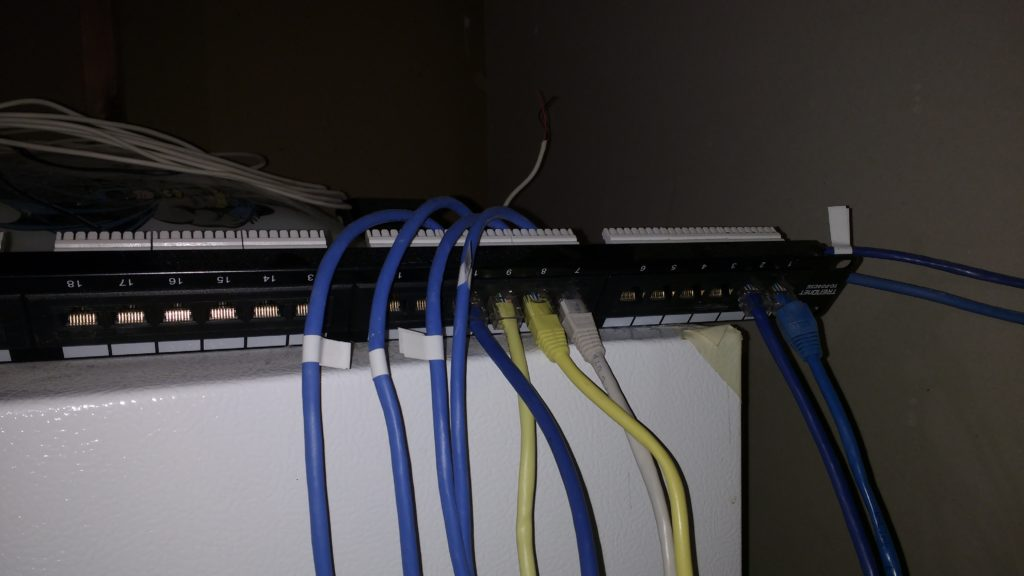and from there to a switch: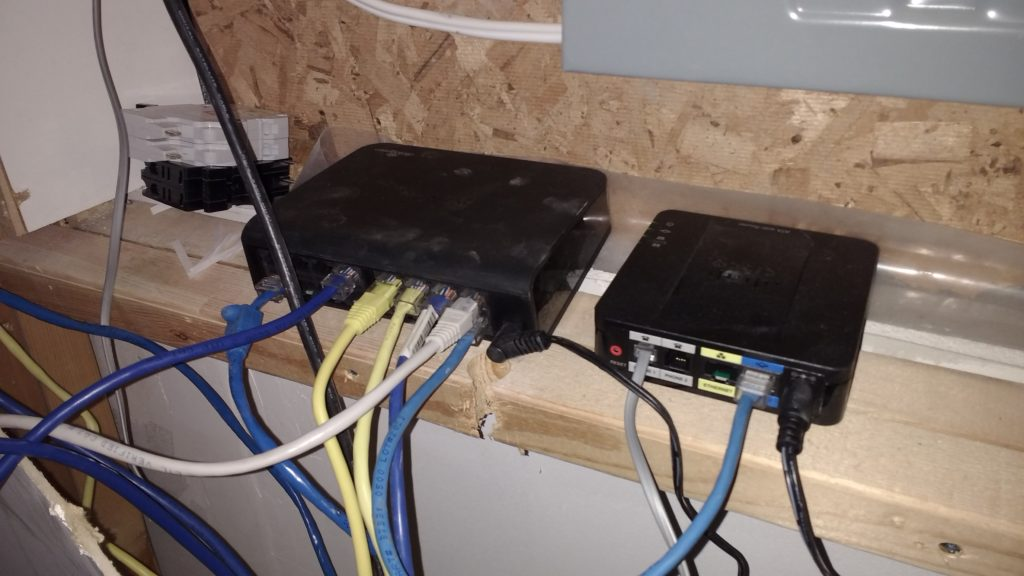One line comes from the router (through the patch panel), and then all the rest of the ethernet lines from the switch go to final destinations. Four of those lines go back to the patch panel and up to the office space. One is plugged into one of the thunderbolt monitors, so I can use a wired connection while “docked”. One goes up to the office space and is connected to an Odroid (which can run the Lz stack on aarch64 hardware). One line goes back to the living room, for optional wired couch surfing, and the last is connected to a voip phone.

I’ve got a lot of finishing touches to do. I plan to mount the patch panel next to the electrical panel, instead of just placing it on top of the freezer down in the laundry room. I’ve also got lines that are running through the ceiling, but are hanging loose still. The wall panel in my office space is currently hanging loose: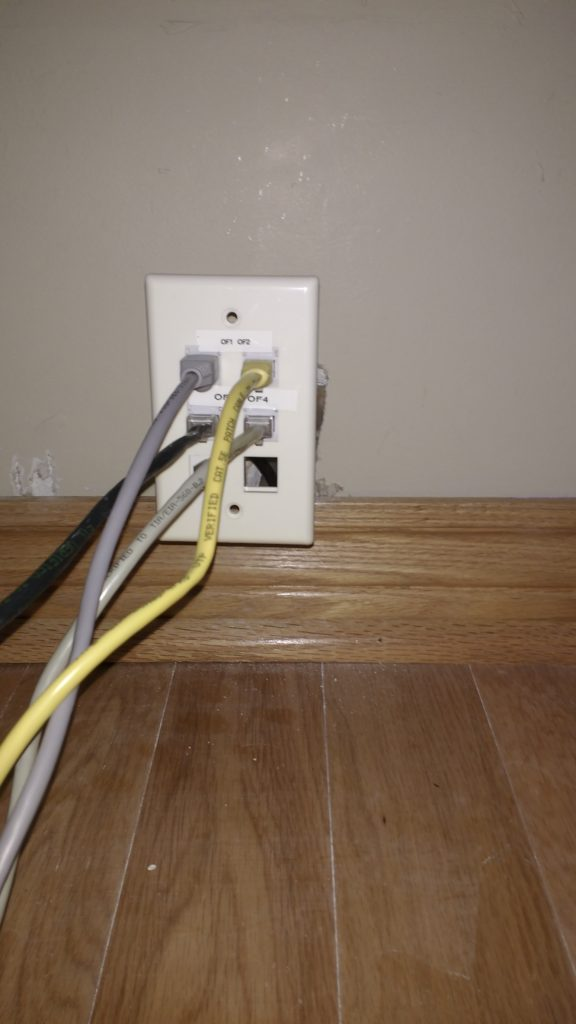I have a low voltage wiring box purchased, but need to cut the hole for it, so I can screw in this little panel and get it nicely out of the way, and do the plastering and painting to fix up the messy fishing holes I made trying to find a route down into the basement.

## M.Eng survey remarks: rant on exams in grad studies

August 28, 2016 Incoherent ramblings

I was asked to rate my satisfaction of aspects of the M.Eng program I’m taking, so took the opportunity to rant about the insanity of exams:

“As a professional, I find that Exam based courses are very artificial, and not terribly meaningful. As an undergrad I did not question this practice, but had forgotten about those days. It was a rather rude shock to see this dark ages practise still in use for graduate studies.

Nowhere in a professional context does it make sense to try to memorize enough content that you can barf out half-ass material sufficient to pass examination questions, or attempt to gain maximum marks in a minimum amount of time. Professional work needs to be complete and accurate. Mistakes cause millions of dollars, and get people fired.

It makes no sense to take any examination, and not be given the opportunity to see the marked exam content. One ought to have the chance to review that material, correcting all deficiencies in one’s understanding. If mistakes are made in a professional context, there is a feedback cycle, where root causes are addressed. This is completely missing from the brain dead process of final exams.

Thankfully, not all the graduate courses I took still used the childish approach of grading based on examinations, but enough did that it was annoying. The aim should not be to produce a mark, but to produce full understanding of the subject material, and demonstrate the ability to apply that material.”

## Shipping with DHL. They will screw you, but not quite as bad as UPS.

August 27, 2016 Incoherent ramblings, Uncategorized , , , , ,

I previously complained about UPS customs clearing charges that I was slammed with receiving back some of my own goods.

Basically, the Canadian government grants shipping companies the right to extort receivers at the point of customs clearing. Canada might add a few cents or a buck or two of tax, but the shipping company is then able to add fees that are orders of magnitude higher than the actual taxes.

I actually stopped buying anything from the United States because of this, and have been buying from Europe and India instead, where I had not yet gotten blasted with customs clearing fees for the items I’ve been buying (usually textbooks).

However, it appears that my luck has run out.  Here’s the newest example, with a $15 dollar clearing fee that DHL added onto about a dollar of tax: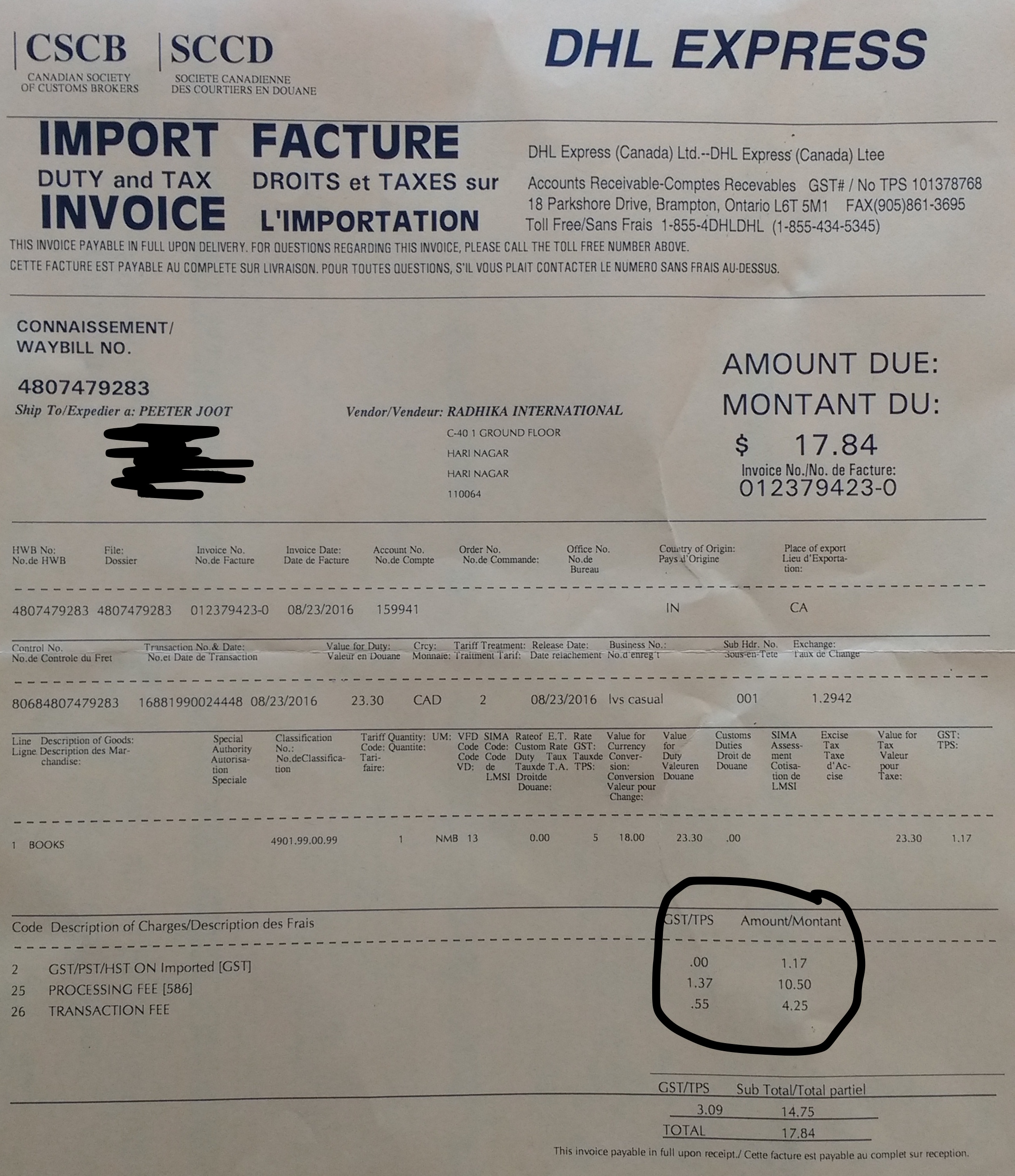Note that I did not pick the shipping company. That was selected by the book seller (one of the abebooks.com resellers). For$1.17 of taxes, DHL has charged me $14.75 of fees, all for the right to allow Canada revenue to steal from me. To add insult to injury, DHL is allowed to charge GST for their extortion service, so I end up paying an additional$3.09 (close to 3x the initial tax amount).  The value of the book + shipping that I purchased was only $23.30! Aside: Why is the GST on$14.75 that high?  I thought that’s a 13% tax, so shouldn’t it be \$1.92?

I’ve found some instructions that explain some of the black magic required to do my own customs clearing:

One of the first steps is to find the CBSA office that I can submit such a clearing form to.  I can narrow that search down to province, but some of these offices are restricted to specific purposes, and it isn’t obvious which of these offices I should use.  For example the one at Buttonville airport appears to be restricted to handling just the cargo that arrives there.

I wonder if there are any local resellers that import used and cheap textbooks in higher quantities and then resell them locally (taking the customs clearing charge only once per shipment)?

## Electric field due to spherical shell

Here’s a problem (2.7) from , to calculate the field due to a spherical shell. The field is

\begin{equation}\label{eqn:griffithsEM2_7:20}
\BE = \frac{\sigma}{4 \pi \epsilon_0} \int \frac{(\Br – \Br’)}{\Abs{\Br – \Br’}^3} da’,
\end{equation}

where $$\Br’$$ is the position to the area element on the shell. For the test position, let $$\Br = z \Be_3$$. We need to parameterize the area integral. A complex-number like geometric algebra representation works nicely.

\begin{equation}\label{eqn:griffithsEM2_7:40}
\begin{aligned}
\Br’
&= R \lr{ \sin\theta \cos\phi, \sin\theta \sin\phi, \cos\theta } \\
&= R \lr{ \Be_1 \sin\theta \lr{ \cos\phi + \Be_1 \Be_2 \sin\phi } + \Be_3 \cos\theta } \\
&= R \lr{ \Be_1 \sin\theta e^{i\phi} + \Be_3 \cos\theta }.
\end{aligned}
\end{equation}

Here $$i = \Be_1 \Be_2$$ has been used to represent to horizontal rotation plane.

The difference in position between the test vector and area-element is

\begin{equation}\label{eqn:griffithsEM2_7:60}
\Br – \Br’
= \Be_3 \lr{ z – R \cos\theta } – R \Be_1 \sin\theta e^{i \phi},
\end{equation}

with an absolute squared length of

\begin{equation}\label{eqn:griffithsEM2_7:80}
\begin{aligned}
\Abs{\Br – \Br’ }^2
&= \lr{ z – R \cos\theta }^2 + R^2 \sin^2\theta \\
&= z^2 + R^2 – 2 z R \cos\theta.
\end{aligned}
\end{equation}

As a side note, this is a kind of fun way to prove the old “cosine-law” identity. With that done, the field integral can now be expressed explicitly

\begin{equation}\label{eqn:griffithsEM2_7:100}
\begin{aligned}
\BE
&= \frac{\sigma}{4 \pi \epsilon_0} \int_{\phi = 0}^{2\pi} \int_{\theta = 0}^\pi R^2 \sin\theta d\theta d\phi
\frac{\Be_3 \lr{ z – R \cos\theta } – R \Be_1 \sin\theta e^{i \phi}}
{
\lr{z^2 + R^2 – 2 z R \cos\theta}^{3/2}
} \\
&= \frac{2 \pi R^2 \sigma \Be_3}{4 \pi \epsilon_0} \int_{\theta = 0}^\pi \sin\theta d\theta
\frac{z – R \cos\theta}
{
\lr{z^2 + R^2 – 2 z R \cos\theta}^{3/2}
} \\
&= \frac{2 \pi R^2 \sigma \Be_3}{4 \pi \epsilon_0} \int_{\theta = 0}^\pi \sin\theta d\theta
\frac{ R( z/R – \cos\theta) }
{
(R^2)^{3/2} \lr{ (z/R)^2 + 1 – 2 (z/R) \cos\theta}^{3/2}
} \\
&= \frac{\sigma \Be_3}{2 \epsilon_0} \int_{u = -1}^{1} du
\frac{ z/R – u}
{
\lr{1 + (z/R)^2 – 2 (z/R) u}^{3/2}
}.
\end{aligned}
\end{equation}

Observe that all the azimuthal contributions get killed. We expect that due to the symmetry of the problem. We are left with an integral that submits to Mathematica, but doesn’t look fun to attempt manually. Specifically

\begin{equation}\label{eqn:griffithsEM2_7:120}
\int_{-1}^1 \frac{a-u}{\lr{1 + a^2 – 2 a u}^{3/2}} du
=
\left\{
\begin{array}{l l}
\frac{2}{a^2} & \quad \mbox{if $$a > 1$$ } \\
0 & \quad \mbox{if $$a < 1$$ } \end{array} \right., \end{equation} so \begin{equation}\label{eqn:griffithsEM2_7:140} \boxed{ \BE = \left\{ \begin{array}{l l} \frac{\sigma (R/z)^2 \Be_3}{\epsilon_0} & \quad \mbox{if $$z > R$$ } \\
0 & \quad \mbox{if $$z < R$$ } \end{array} \right. } \end{equation} In the problem, it is pointed out to be careful of the sign when evaluating $$\sqrt{ R^2 + z^2 - 2 R z }$$, however, I don't see where that is even useful?

# References

 David Jeffrey Griffiths and Reed College. Introduction to electrodynamics. Prentice hall Upper Saddle River, NJ, 3rd edition, 1999.